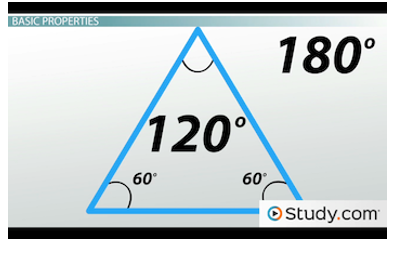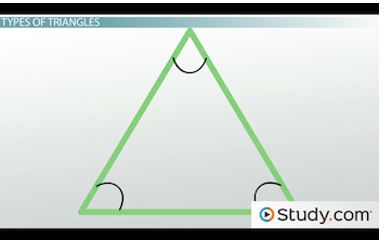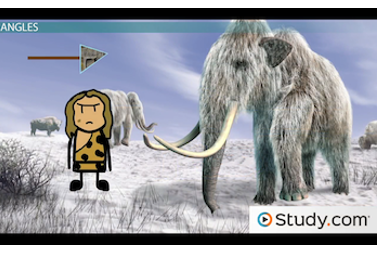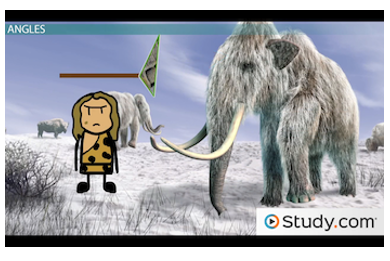# Properties of Shapes: Triangles

#### Video Lesson on Properties of Shapes: Triangles

This lesson video Not available at this time available video coming soon

### Properties of Shapes: Triangles

Isosceles, equilateral and obtuse, oh my! These are terms for triangles. But, what do they mean? In this lesson, we'll explore the properties of triangles and take a closer look at the different types of triangles you may encounter.

### Seeing Triangles

Triangles - they're the three-sided shape you see everywhere you look. They're the symbol for play and the sign to slow down. They're the ears on a cat and a slice of pizza. They're the gateway to the unknown and the monument to a long-ago empire.

They're even the musical instrument calling us to dinner if we live on a 19th century farm. Though I don't know how you're watching this online video from a 19th century farm. Let's take a closer look at the many facets of this seemingly simple shape.

### Basic Properties

First, let's define what we're talking about. A triangle is a polygon with three sides and three angles. You can remember that triangles have three sides and angles by that 'tri' in the name. 'Tri' means 'three,' whether you're talking about geometry, tricycles or triceratops.

In a triangle, the interior angles always add up to 180 degrees. So, in the triangle below, if one angle is 60 and another is 60, which is 120 total, then the other one has to be 180 - 120, which is also 60. If you ever know two angles of a triangle, you can always find the third by remembering the 180-degree rule.If you know two angles of a triangle, you can determine the third.

### Types of Triangles

Triangles that have particular properties get their own names. For example, this one:In an equilateral triangle, all the sides and angles are equal<,/p>

Notice that all the angles are the same. As you might guess, there's a direct correlation between the angles and sides. When the angles are all the same, so are the sides. So, this is an example of an equilateral triangle, which is a triangle with three equal sides and three equal angles. Do you hear 'equal' in 'equilateral'? The term literally means 'equal sides.'

Some triangles only have two equal sides. A triangle with two equal sides and two equal angles is called an isosceles triangle. I think of isosceles triangles as an equilateral triangle's younger sibling. Its equalness is not as complete. Note below that the equal sides are always opposite the equal angles. So, if the angles on the bottom are equal, the sides with blue arrows are equal.Isosceles triangles have two equal sides and two equal angles.

Then there's the awkward cousin to equilateral and isosceles triangles: the scalene triangle. A scalene triangle is a triangle with no equal sides or angles. Scalene means unequal, but it doesn't sound like unequal. It sounds like 'scale.' Think about yourself on a scale. If you're like me, you probably don't weigh exactly what you'd like to weigh. You're a bit (or more than a bit) off. And, scalene triangles are out of balance, too. None of their sides match.

### Angles

We just compared triangles by their sides and angles, but we also classify triangles by just their angles. For example, a triangle with a right angle, which is a 90-degree angle, is called a right triangle. That makes sense, right? Right angle - right triangle.

But, what if you have a triangle with all angles less than 90 degrees? That's an acute triangle. The opposite of this is a triangle with an angle that's more than 90 degrees. That's an obtuse triangle. You may know that an angle less than 90 degrees is acute and an angle more than 90 degrees is obtuse. That's where these triangle names originate. You can also think of it this way: Acute means 'sharp or pointed.' Think of an acute pain. Or, forget the Latin derivation and just think of what makes 'a cute' triangle. Small angles!

Conversely, obtuse means 'dull or blunt.' Let's imagine we're early humans making arrowheads to kill some woolly mammoths. If your arrowhead looks like the one below - acute triangles - you might feast on mammoth steaks tonight. Mmm, gamey.Acute triangles have angles less than 90 degrees.

If they look like this one below - obtuse - they're duller, less pointy, and that mammoth may feast on you. Mmm, trampled to death.Obtuse triangles have an angle greater than 90 degrees.

### Combining Names

These names for triangles are not mutually exclusive. For example, an equilateral triangle is necessarily an acute triangle. Think about that. All equilateral triangle angles are 60 degrees, so they're all less than 90 degrees. Also, you can have an isosceles triangle that's also a right triangle.

If one angle is 90 degrees and it's an isosceles triangle, the other angles must add up to 90, so they're each 45 degrees. Scalene triangles can be acute, obtuse or right triangles. That seems fitting since most people feel out of balance on a scale.

### Lesson Summary

In summary, we learned about triangles, which are polygons with three sides and three angles. We looked at three main types of triangles. First, equilateral triangles are triangles with three equal sides and angles. Second, isosceles triangles are triangles with two equal sides and angles. Third, the awkward cousin, scalene triangles, which are triangles with no equal sides or angles.

Then we looked at the angles of triangles. There are right triangles, or triangles with one right angle. Then there are acute triangles, where all angles are less than 90 degrees, and obtuse triangles, where one angle is more than 90 degrees. Now I think I hear a triangle being played. It must be time for a 19th century farm dinner.

### More Educational and Fun StuffMore In-depth knowledge about what you need. Detailed about test preparation, English Writing, TOEFL, and IELTS.